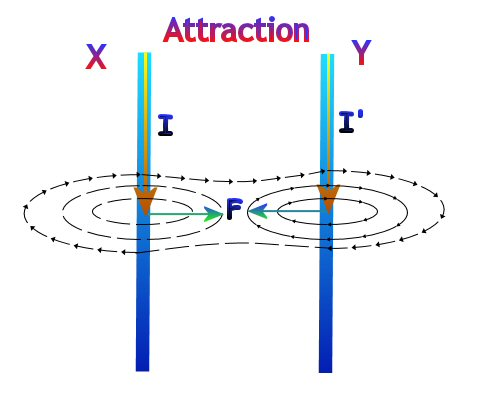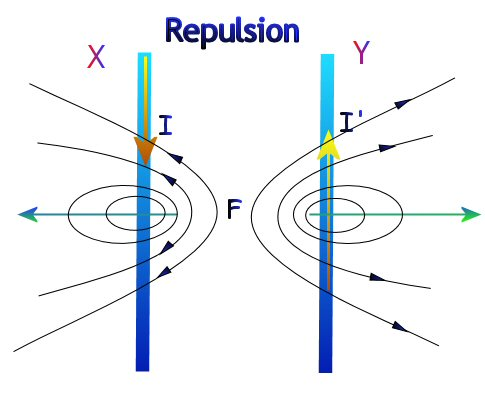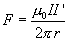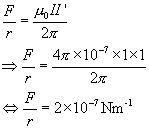## -Force, Field and Current-

When playing with magnets we've all experienced the force associated with magnetic fields. I'm sure you've tried to bring together like poles of bar magnets just to feel the force of repulsion they exert on each other. Perhaps, like me you've used magnets to make  toy cars apparently move on their own to amaze your younger relatives.

Lets extend our investigations of magnetic attraction and repulsion to current carrying conductors.

### Force between two Current Carrying Conductors in ParallelWhen two parallel conductors carry current in the same direction their associated magnetic fields interact creating an attractive force between the conductors. (Notice the reduced flux density between the conductors.) The resulting lines of flux can be visualized as pulling the conductors together. (Verify the direction of the field  by using the Corkscrew rule)When the current flows are in opposite directions the magnetic field between the conductors is reinforced. This causes the conductors to repel each other.

Consider the diagram above. The line of flux midway between the conductors X and Y points outwards (towards you, the reader) It is in the horizontal plane as the conductors X and Y are in the vertical plane. As Fleming's left hand rule will show this results in a horizontal repulsive force between the conductors.

#### Important Points

• Force between parallel conductors carrying current in the same direction is attractive (AKA Like currents Attract)
• Force between parallel conductors carrying current in the opposite direction is repulsive (AKA Unlike Currents Repel)

The magnitude of the force between the two conductors depends on several variables. It should be fairly obvious that increasing the current or decreasing the distance between the conductors will result in an increased force between the conductors.

#### Magnitude of the Force - Optional

The force per meter length, F, on X (or Y) is given by,where
I = current in X
I' = current in Y
r = perpendicular distance between X and Y
m0 = permeability of free space (4π * 10-7 Henry meter -1)

##### Example

Calculate the force per meter between two long thin parallel silver wires separated by a distance of 1 m when each carry a current of 1A in the same direction.

Solution: Using the above formula,